# fmesh

## Syntax

``fmesh(f)``
``fmesh(f,[min max])``
``````fmesh(f,[xmin xmax ymin ymax])``````
``fmesh(funx,funy,funz)``
``````fmesh(funx,funy,funz,[uvmin uvmax])``````
``````fmesh(funx,funy,funz,[umin umax vmin vmax])``````
``fmesh(___,LineSpec)``
``fmesh(___,Name,Value)``
``fmesh(ax,___)``
``obj = fmesh(___)``

## Description

example

````fmesh(f)` creates a mesh plot of the symbolic expression `f(x,y)` over the default interval `[-5 5]` for `x` and `y`.```
````fmesh(f,[min max])` plots `f(x,y)` over the interval `[min max]` for `x` and `y`.```

example

``````fmesh(f,[xmin xmax ymin ymax])``` plots `f(x,y)` over the interval `[xmin xmax]` for `x` and `[ymin ymax]` for `y`. The `fmesh` function uses `symvar` to order the variables and assign intervals.```

example

````fmesh(funx,funy,funz)` plots the parametric mesh `x = x(u,v)`, `y = y(u,v)`, ```z = z(u,v)``` over the interval `[-5 5]` for `u` and `v`.```
``````fmesh(funx,funy,funz,[uvmin uvmax])``` plots the parametric mesh ```x = x(u,v)```, `y = y(u,v)`, `z = z(u,v)` over the interval `[uvmin uvmax]` for `u` and `v`.```
``````fmesh(funx,funy,funz,[umin umax vmin vmax])``` plots the parametric mesh `x = x(u,v)`, ```y = y(u,v)```, `z = z(u,v)` over the interval `[umin umax]` for `u` and ```[vmin vmax]``` for `v`. The `fmesh` function uses `symvar` to order the parametric variables and assign intervals.```
````fmesh(___,LineSpec)` uses the `LineSpec` to set the line style, marker symbol, and plot color.```
````fmesh(___,Name,Value)` specifies surface properties using one or more `Name,Value` pair arguments. Use this option with any of the input argument combinations in the previous syntaxes.```
````fmesh(ax,___)` plots into the axes with the object `ax` instead of the current axes object `gca`.```
````obj = fmesh(___)` returns a function surface object or a parameterized function surface object. Use the object to query and modify properties of a specific mesh.```

## Examples

collapse all

Plot a mesh of the input $\mathrm{sin}\left(x\right)+\mathrm{cos}\left(y\right)$ over the default range $-5 and $-5.

```syms x y fmesh(sin(x)+cos(y))```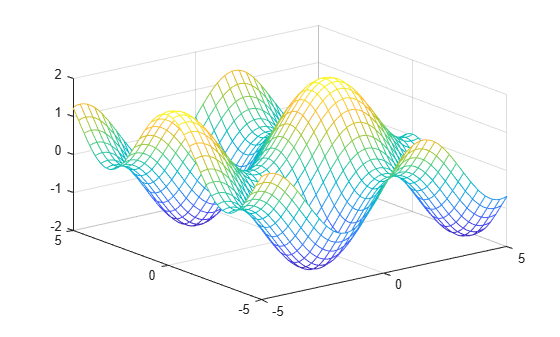Plot a 3-D mesh of the real part of ${tan}^{-1}\left(x+iy\right)$ over the default range $-5 and $-5.

```syms f(x,y) f(x,y) = real(atan(x + i*y)); fmesh(f)```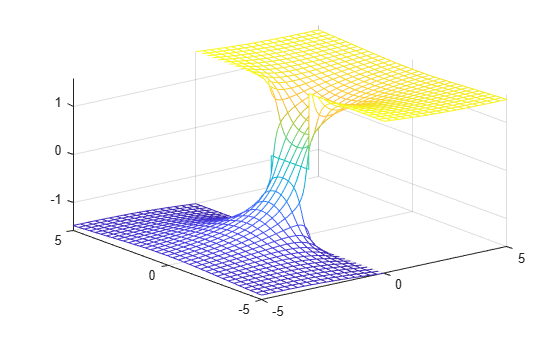Plot $\mathrm{sin}\left(x\right)+\mathrm{cos}\left(y\right)$ over $-\pi and $-5 by specifying the plotting interval as the second argument of `fmesh`.

```syms x y f = sin(x) + cos(y); fmesh(f, [-pi pi -5 5])```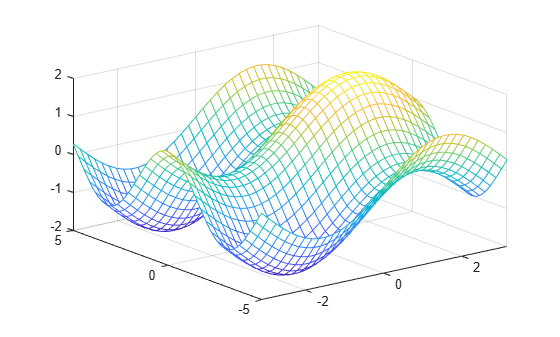Plot the parameterized mesh

`$\begin{array}{c}x=r\mathrm{cos}\left(s\right)\mathrm{sin}\left(t\right)\\ y=r\mathrm{sin}\left(s\right)\mathrm{sin}\left(t\right)\\ z=r\mathrm{cos}\left(t\right)\\ where\phantom{\rule{1em}{0ex}}r=8+\mathrm{sin}\left(7s+5t\right)\end{array}$`

for $0 and $0. Make the aspect ratio of the axes equal using `axis equal`. See the entire mesh by making the mesh partially transparent using `alpha`.

```syms s t r = 8 + sin(7*s + 5*t); x = r*cos(s)*sin(t); y = r*sin(s)*sin(t); z = r*cos(t); fmesh(x, y, z, [0 2*pi 0 pi], 'Linewidth', 2) axis equal alpha(0.8)```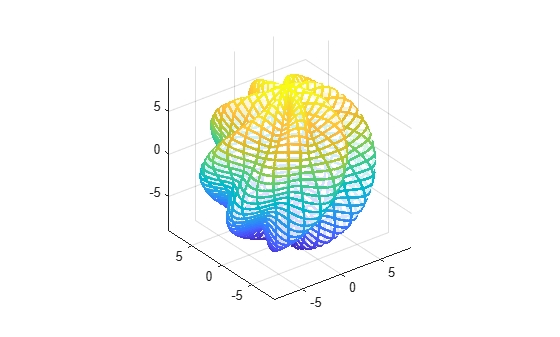## Input Arguments

collapse all

Expression or function to be plotted, specified as a symbolic expression or function.

Plotting interval for x- and y-axes, specified as a vector of two numbers. The default is `[-5 5]`.

Plotting interval for x- and y-axes, specified as a vector of four numbers. The default is `[-5 5 -5 5]`.

Parametric functions of `u` and `v`, specified as a symbolic expression or function.

Plotting interval for `u` and `v` axes, specified as a vector of two numbers. The default is ```[-5 5]```.

Plotting interval for `u` and `v`, specified as a vector of four numbers. The default is ```[-5 5 -5 5]```.

Axes object. If you do not specify an axes object, then `fmesh` uses the current axes.

Line style, marker, and color, specified as a string scalar or character vector containing symbols. The symbols can appear in any order. You do not need to specify all three characteristics (line style, marker, and color). For example, if you omit the line style and specify the marker, then the plot shows only the marker and no line.

Example: `"--or"` is a red dashed line with circle markers.

Line StyleDescriptionResulting Line
`"-"`Solid line`"--"`Dashed line`":"`Dotted line`"-."`Dash-dotted lineMarkerDescriptionResulting Marker
`"o"`Circle`"+"`Plus sign`"*"`Asterisk`"."`Point`"x"`Cross`"_"`Horizontal line`"|"`Vertical line`"square"`Square`"diamond"`Diamond`"^"`Upward-pointing triangle`"v"`Downward-pointing triangle`">"`Right-pointing triangle`"<"`Left-pointing triangle`"pentagram"`Pentagram`"hexagram"`HexagramColor NameShort NameRGB TripletAppearance
`"red"``"r"``[1 0 0]``"green"``"g"``[0 1 0]``"blue"``"b"``[0 0 1]``"cyan"` `"c"``[0 1 1]``"magenta"``"m"``[1 0 1]``"yellow"``"y"``[1 1 0]``"black"``"k"``[0 0 0]``"white"``"w"``[1 1 1]`### Name-Value Arguments

Specify optional pairs of arguments as `Name1=Value1,...,NameN=ValueN`, where `Name` is the argument name and `Value` is the corresponding value. Name-value arguments must appear after other arguments, but the order of the pairs does not matter.

Before R2021a, use commas to separate each name and value, and enclose `Name` in quotes.

Example: `'Marker','o','MarkerFaceColor','red'`

Number of evaluation points per direction, specified as a number. The default is `35`. Because `fmesh` objects use adaptive evaluation, the actual number of evaluation points is greater.

Example: `100`

Display contour plot under plot, specified as `'on'` or `'off'`, or as numeric or logical `1` (`true`) or `0` (`false`). A value of `'on'` is equivalent to true, and `'off'` is equivalent to `false`. Thus, you can use the value of this property as a logical value. The value is stored as an on/off logical value of type `matlab.lang.OnOffSwitchState`.

Line color, specified as `'interp'`, an RGB triplet, a hexadecimal color code, a color name, or a short name. The default value of `'interp'` colors the edges based on the `ZData` property values.

For a custom color, specify an RGB triplet or a hexadecimal color code.

• An RGB triplet is a three-element row vector whose elements specify the intensities of the red, green, and blue components of the color. The intensities must be in the range `[0,1]`, for example, ```[0.4 0.6 0.7]```.

• A hexadecimal color code is a string scalar or character vector that starts with a hash symbol (`#`) followed by three or six hexadecimal digits, which can range from `0` to `F`. The values are not case sensitive. Therefore, the color codes `"#FF8800"`, `"#ff8800"`, `"#F80"`, and `"#f80"` are equivalent.

Alternatively, you can specify some common colors by name. This table lists the named color options, the equivalent RGB triplets, and hexadecimal color codes.

Color NameShort NameRGB TripletHexadecimal Color CodeAppearance
`"red"``"r"``[1 0 0]``"#FF0000"``"green"``"g"``[0 1 0]``"#00FF00"``"blue"``"b"``[0 0 1]``"#0000FF"``"cyan"` `"c"``[0 1 1]``"#00FFFF"``"magenta"``"m"``[1 0 1]``"#FF00FF"``"yellow"``"y"``[1 1 0]``"#FFFF00"``"black"``"k"``[0 0 0]``"#000000"``"white"``"w"``[1 1 1]``"#FFFFFF"``"none"`Not applicableNot applicableNot applicableNo color

Here are the RGB triplets and hexadecimal color codes for the default colors MATLAB® uses in many types of plots.

`[0 0.4470 0.7410]``"#0072BD"``[0.8500 0.3250 0.0980]``"#D95319"``[0.9290 0.6940 0.1250]``"#EDB120"``[0.4940 0.1840 0.5560]``"#7E2F8E"``[0.4660 0.6740 0.1880]``"#77AC30"``[0.3010 0.7450 0.9330]``"#4DBEEE"``[0.6350 0.0780 0.1840]``"#A2142F"`Example: `'blue'`

Example: ```[0 0 1]```

Example: `'#0000FF'`

Line style, specified as one of the options listed in this table.

Line StyleDescriptionResulting Line
`"-"`Solid line`"--"`Dashed line`":"`Dotted line`"-."`Dash-dotted line`"none"`No lineNo line

Line width, specified as a positive value in points, where 1 point = 1/72 of an inch. If the line has markers, then the line width also affects the marker edges.

The line width cannot be thinner than the width of a pixel. If you set the line width to a value that is less than the width of a pixel on your system, the line displays as one pixel wide.

Marker symbol, specified as one of the values listed in this table. By default, the object does not display markers. Specifying a marker symbol adds markers at each data point or vertex.

MarkerDescriptionResulting Marker
`"o"`Circle`"+"`Plus sign`"*"`Asterisk`"."`Point`"x"`Cross`"_"`Horizontal line`"|"`Vertical line`"square"`Square`"diamond"`Diamond`"^"`Upward-pointing triangle`"v"`Downward-pointing triangle`">"`Right-pointing triangle`"<"`Left-pointing triangle`"pentagram"`Pentagram`"hexagram"`Hexagram`"none"`No markersNot applicable

Marker outline color, specified as `'auto'`, an RGB triplet, a hexadecimal color code, a color name, or a short name. The default value of `'auto'` uses the same color as the `EdgeColor` property.

For a custom color, specify an RGB triplet or a hexadecimal color code.

• An RGB triplet is a three-element row vector whose elements specify the intensities of the red, green, and blue components of the color. The intensities must be in the range `[0,1]`, for example, ```[0.4 0.6 0.7]```.

• A hexadecimal color code is a string scalar or character vector that starts with a hash symbol (`#`) followed by three or six hexadecimal digits, which can range from `0` to `F`. The values are not case sensitive. Therefore, the color codes `"#FF8800"`, `"#ff8800"`, `"#F80"`, and `"#f80"` are equivalent.

Alternatively, you can specify some common colors by name. This table lists the named color options, the equivalent RGB triplets, and hexadecimal color codes.

Color NameShort NameRGB TripletHexadecimal Color CodeAppearance
`"red"``"r"``[1 0 0]``"#FF0000"``"green"``"g"``[0 1 0]``"#00FF00"``"blue"``"b"``[0 0 1]``"#0000FF"``"cyan"` `"c"``[0 1 1]``"#00FFFF"``"magenta"``"m"``[1 0 1]``"#FF00FF"``"yellow"``"y"``[1 1 0]``"#FFFF00"``"black"``"k"``[0 0 0]``"#000000"``"white"``"w"``[1 1 1]``"#FFFFFF"``"none"`Not applicableNot applicableNot applicableNo color

Here are the RGB triplets and hexadecimal color codes for the default colors MATLAB uses in many types of plots.

`[0 0.4470 0.7410]``"#0072BD"``[0.8500 0.3250 0.0980]``"#D95319"``[0.9290 0.6940 0.1250]``"#EDB120"``[0.4940 0.1840 0.5560]``"#7E2F8E"``[0.4660 0.6740 0.1880]``"#77AC30"``[0.3010 0.7450 0.9330]``"#4DBEEE"``[0.6350 0.0780 0.1840]``"#A2142F"`Example: `[0.5 0.5 0.5]`

Example: `'blue'`

Example: `'#D2F9A7'`

Marker fill color, specified as `'auto'`, an RGB triplet, a hexadecimal color code, a color name, or a short name. The `'auto'` value uses the same color as the `MarkerEdgeColor` property.

For a custom color, specify an RGB triplet or a hexadecimal color code.

• An RGB triplet is a three-element row vector whose elements specify the intensities of the red, green, and blue components of the color. The intensities must be in the range `[0,1]`, for example, ```[0.4 0.6 0.7]```.

• A hexadecimal color code is a string scalar or character vector that starts with a hash symbol (`#`) followed by three or six hexadecimal digits, which can range from `0` to `F`. The values are not case sensitive. Therefore, the color codes `"#FF8800"`, `"#ff8800"`, `"#F80"`, and `"#f80"` are equivalent.

Alternatively, you can specify some common colors by name. This table lists the named color options, the equivalent RGB triplets, and hexadecimal color codes.

Color NameShort NameRGB TripletHexadecimal Color CodeAppearance
`"red"``"r"``[1 0 0]``"#FF0000"``"green"``"g"``[0 1 0]``"#00FF00"``"blue"``"b"``[0 0 1]``"#0000FF"``"cyan"` `"c"``[0 1 1]``"#00FFFF"``"magenta"``"m"``[1 0 1]``"#FF00FF"``"yellow"``"y"``[1 1 0]``"#FFFF00"``"black"``"k"``[0 0 0]``"#000000"``"white"``"w"``[1 1 1]``"#FFFFFF"``"none"`Not applicableNot applicableNot applicableNo color

Here are the RGB triplets and hexadecimal color codes for the default colors MATLAB uses in many types of plots.

`[0 0.4470 0.7410]``"#0072BD"``[0.8500 0.3250 0.0980]``"#D95319"``[0.9290 0.6940 0.1250]``"#EDB120"``[0.4940 0.1840 0.5560]``"#7E2F8E"``[0.4660 0.6740 0.1880]``"#77AC30"``[0.3010 0.7450 0.9330]``"#4DBEEE"``[0.6350 0.0780 0.1840]``"#A2142F"`Example: `[0.3 0.2 0.1]`

Example: `'green'`

Example: `'#D2F9A7'`

Marker size, specified as a positive value in points, where 1 point = 1/72 of an inch.

## Output Arguments

collapse all

One or more objects, returned as a scalar or a vector. The object is either a function surface object or parameterized mesh object, depending on the type of plot. You can use these objects to query and modify properties of a specific line. For details, see FunctionSurface Properties and ParameterizedFunctionSurface Properties.

## Tips

• For additional examples, follow the `fsurf` page because `fmesh` and `fsurf` share the same syntax. All examples on the `fsurf` page apply to `fmesh`.

## Algorithms

`fmesh` assigns the symbolic variables in `f` to the x-axis, then the y-axis, and `symvar` determines the order of the variables to be assigned. Therefore, variable and axis names might not correspond. To force `fmesh` to assign x or y to its corresponding axis, create the symbolic function to plot, then pass the symbolic function to `fmesh`.

For example, the following code plots the mesh of f(x,y) = sin(y) in two ways. The first way forces the waves to oscillate with respect to the y-axis. In other words, the first plot assigns the y variable to the corresponding y-axis. The second plot assigns y to the x-axis because it is the first (and only) variable in the symbolic function.

```syms x y; f(x,y) = sin(y); figure; subplot(2,1,1) fmesh(f); subplot(2,1,2) fmesh(f(x,y)); % Or fmesh(sin(y)); ```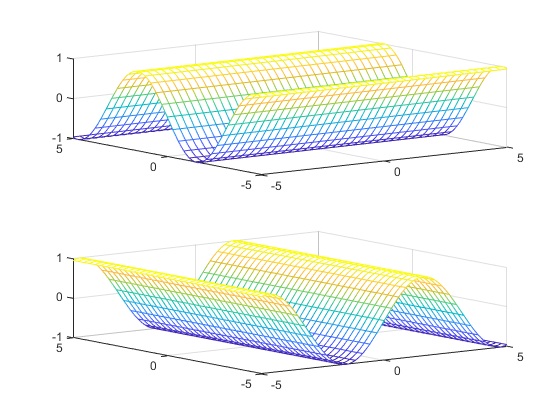## Version History

Introduced in R2016a